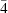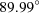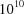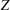# issue contentsFOUNDATIONSADVANCES
ISSN: 2053-2733

# November 2001 issueCover illustration: Graphical representation of density modification due to third-order terms b113 = -b223 (point group) in the Gram-Charlier series expansion of a Gaussian atomic probability density function. Courtesy of W. F. Kuhs.

## research papers

### Systematic intensity errors caused by spectral truncation: origin and remedy

X-ray absorption by the monochromator determines the wavelength dispersion in the monochromated X-ray beam and explains the Δλλ values of 0.03, 0.14 and 0.16 for graphite(002)-monochromated Cu, Mo and Rh radiation. To eliminate the systematic intensity truncation errors, caused by the large wavelength dispersion, a correction is proposed based on the applied scan angle and the real beam spectrum.

### Chirality-induced `forbidden' reflections in X-ray resonant scattering

It is shown that additional Bragg reflections can appear in resonant near-edge X-ray diffraction exclusively owing to the local chirality associated with the left–right asymmetric environment of scattering atoms. This effect occurs even in centrosymmetric crystals, which are not gyrotropic in optics, and some realistic examples are considered in detail (α-Fe2O3, LiNbO3 etc.).

### Coincidence site lattice theory of multicrystalline ensembles

The coincidence site lattice theory is extended to multicrystalline ensembles, such as quadruple points and multiple junctions of grains.

### Relativistic analytical wave functions and scattering factors for neutral atoms beyond Kr and for all chemically important ions up to I−

Relativistic wave functions for elements with Z = 37–54 have been fitted with a linear combination of Slater-type functions for use in charge-density analysis. Numerical relativistic wave functions have been calculated for all chemically relevant ions up to Z = 54, and corresponding analytical espressions have been derived. The calculated X-ray scattering factors are parameterized with six Gausian functions. Results are available at http://harker.chem.buffalo.edu.

### Electron-density distribution in stishovite, SiO2: a new high-energy synchrotron-radiation study

100 keV synchrotron radiation was used to obtain essentially absorption- and extinction-free data for the refinement of a multipole model electron-density distribution and ensuing topological analysis. Results are compared with ab initio density functional theory calculations and correlated with Si—O bond properties from geometry-optimized hydroxyacid molecules.

### A simple inverse method for calculating electron-density maps

This paper describes a real-space reconstruction method for calculating electron-density maps.

### A fast Newton method for entropy maximization in statistical phase estimation

A fast Newton method is developed to solve the entropy maximization problem in the Bayesian statistical approach to phase estimation. The method requires only O(n log n) instead of standard O(n3) floating point operations per iteration, while converging in the same rate as the standard Newton method.

### Is the border surface of a crystal indeed the weakest point of the dynamical theory of diffraction?

In Laue's dynamical theory of diffraction, the boundary conditions claim to introduce a mathematical plane instead of the discrete atomic surface of the crystal. This assumption is analyzed from the point of view of Ewald's theory based on the microscopic discrete model of a crystal, where no boundary conditions are needed.

### A new phasing method based on the principle of minimum charge

A description of a new phasing method and demonstration of its use for crystal and quasicrystal structure determination are presented.

### The method of joint probability distribution functions applied to MAD techniques. The two-wavelength case for acentric crystals

The joint probability distribution functions method is applied to estimate phases from multiple-wavelength anomalous dispersion (MAD) data in the two-wavelength case for acentric crystals.

### Ultra-high-angle double-crystal X-ray diffrac­tometry (U-HADOX) for determining a change in the lattice spacing: theory

Extension of Bragg angle up tohas been examined theoretically for high-angle double-crystal X-ray diffractometry (HADOX). It is shown that an accuracy of a change in the lattice spacing to one part incan be attained.

### Atomic scattering factors for K-shell electron energy-loss spectroscopy

Atomic scattering factors for-shell electron energy-loss spectroscopy have been calculated for elements in the range= 6 (carbon) to= 50 (tin).

## short communications

### Magnetic space-group types

Opechowski–Guccione symbols for the 1651 magnetic space-group types are uniquely specified independent of International Tables for Crystallography.

### Symmetry relations of magnetic twin laws

Magnetic symmetry groups and magnetic twinning groups of domain pairs are tabulated.

### A modified X − Y method

To solve the crystallographic phase problem, simulated annealing is used to minimize a total cost function with respect to atomic coordinates. The total cost function consists of the X − Y function plus a crystallographic residual.

### C20 to C60 fullerenes: combinatorial types and symmetries

A figure giving the point groups for all combinatorially non-isomorphic C20 to C60 fullerenes (5770 in common) is contributed. The fullerenes of 6 to 120 automorphism group orders (80 in common) are drawn in the Shlegel projections and characterized by the point groups.

### An efficient ab initio calculation of powder diffraction intensity using Debye's equation

A new efficient algorithm is presented for the evaluation of the Debye equation for powder diffraction intensity having applications for achieving direct intensity profile fitting.